# 5 Equation Solutions

Below are solutions to the practice equations. There are multiple ways to express some of the equations, so your solutions do not need to exactly match the answers given.

1.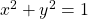[‌latex]x^2 + y^2 = 1[/latex]
2.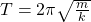[‌latex]T = 2 \pi \sqrt{\frac{m}{k}}[/latex]
3.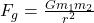[‌latex]F_g = \frac{Gm_1 m_2}{r^2}[/latex]
4.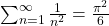[‌latex]\sum_{n=1}^{\infty} \frac{1}{n^2} = \frac{\pi^2}{6}[/latex]
If you want the sum to have the limits above and below, like you see in most textbooks, you can use the command \displaystyle.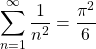[‌latex]\displaystyle \sum_{n=1}^{\infty} \frac{1}{n^2} = \frac{\pi^2}{6}[/latex]
5. The equation is missing a bracket to close the numerator of the fraction, shown in bold below.
[‌latex] x = \frac{-b \pm \sqrt{b^2 + 4ac}}{2a} [/latex]